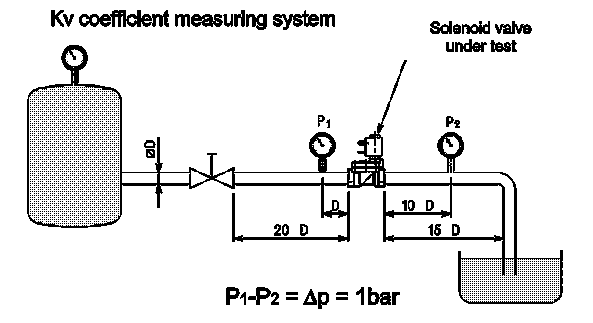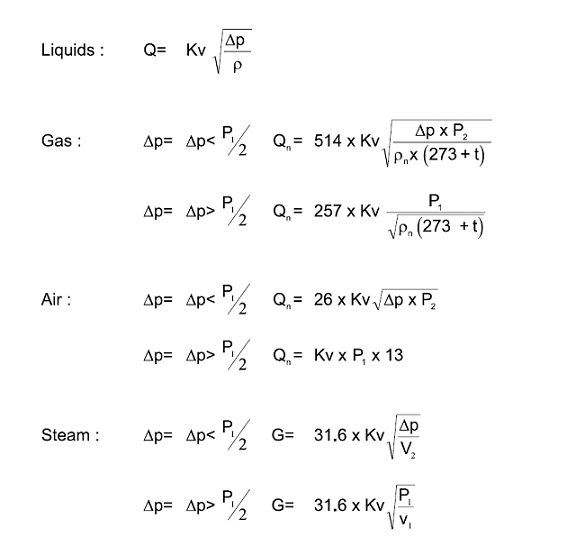## Flow Calculation - (Kv)

By Admin on Fri 10 February 2017

Each solenoid valve has a flow coefficent(Kv). In this article we help explain how to work out your valves KV

Given the loss of flowing pressure (pressure drop), the media type and the working pressure it’s possible to calculate the flow rate.
The flow coefficient is determined by way of experimentation according to the standard VDE 2173 and it represents the quantity of water discharged from the solenoid valve with a pressure difference of 1bar at temperatures between 5°C and 40°C.Kv = m3/h - Flow coefficient
Q = m3/h - Flow
Qn = m3n/h - Normal flow (20°C 760mm Hg)
P1 = bar - Inlet pressure - (Gauge pressure + 1)
P2 = bar - Outlet pressure - (Gauge pressure – 1)
DP = bar - Pressure drop - (Differential pressure between inlet and outlet pressure)
r = Kg/dm³ - Relative density with respect to water (Water at 4°C = 1)
rn = Kg/dm³ - Normal relative density as to the air
G = Kg/h - Mass
t = °C - Inlet media temperature
V1 = m3/Kg - Inlet specific volume
V2 = m3/Kg - Outlet specific volume referred to “P2” pressure and “t” temperature